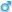###Author Topic: Mathematics  (Read 94 times)

#### Mathesh

• Jr. Member
•• Posts: 47
• Total likes: 5
• Karma: +3/-0
• Gender:##### Mathematics
« on: June 29, 2020, 07:02:57 PM »Mathematics (from Greek: μάθημα, máthēma, 'knowledge, study, learning') includes the study of such topics as quantity (number theory), structure (algebra), space (geometry), and change (mathematical analysis). It has no generally accepted definition.
Mathematicians seek and use patterns to formulate new conjectures; they resolve the truth or falsity of such by mathematical proof. When mathematical structures are good models of real phenomena, mathematical reasoning can be used to provide insight or predictions about nature. Through the use of abstraction and logic, mathematics developed from counting, calculation, measurement, and the systematic study of the shapes and motions of physical objects. Practical mathematics has been a human activity from as far back as written records exist. The research required to solve mathematical problems can take years or even centuries of sustained inquiry.
Rigorous arguments first appeared in Greek mathematics, most notably in Euclid's Elements. Since the pioneering work of Giuseppe Peano (18581932), David Hilbert (18621943), and others on axiomatic systems in the late 19th century, it has become customary to view mathematical research as establishing truth by rigorous deduction from appropriately chosen axioms and definitions. Mathematics developed at a relatively slow pace until the Renaissance, when mathematical innovations interacting with new scientific discoveries led to a rapid increase in the rate of mathematical discovery that has continued to the present day.
Mathematics is essential in many fields, including natural science, engineering, medicine, finance, and the social sciences. Applied mathematics has led to entirely new mathematical disciplines, such as statistics and game theory. Mathematicians engage in pure mathematics (mathematics for its own sake) without having any application in mind, but practical applications for what began as pure mathematics are often discovered later.

Sent from my SM-A205F using Tapatalk

SimplePortal 2.3.7 © 2008-2021, SimplePortal